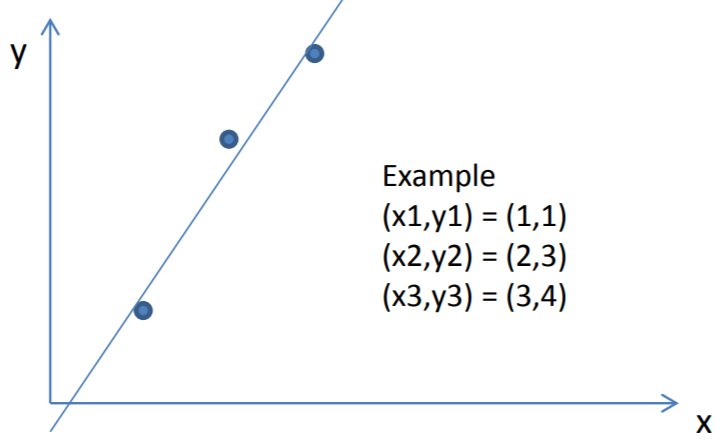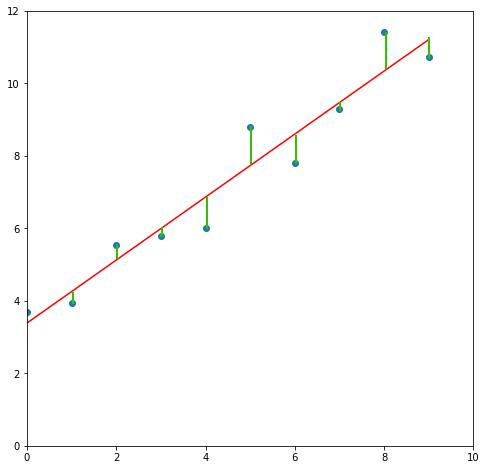• 最小二乘法推导 假设一组数据线性关系为  y=β0+β1x\ y=\beta_0 +\beta_1x y=β0​+β1​x 实际建模中系数 β0,β1\ \beta_0,\beta_1 β0​,β1​是未知的，但是我们的数据是已知的，为了让...
最小二乘法推导
假设一组数据线性关系为 $\ y=\beta_0 +\beta_1x$
实际建模中系数$\ \beta_0,\beta_1$是未知的，但是我们的数据是已知的，为了让我们估计的$\ \hat{y}$与实际的$\ y$ 尽可能接近，我们使
$\ RSS = (y_1-\hat{y}_1)^2+(y_2-\hat{y}_2)^2+……+(y_n-\hat{y}_n)^2$
$\ = (y_1-\beta_0-\beta_1x_1)^2+(y_2-\beta_0-\beta_1x_2)^2+……+(y_2-\beta_0-\beta_1x_2)^2$
$\ =\sum_{i=1}^n(y_i-\beta_0-\beta_1x_i)^2$
因为$\ RSS$最小值为0，没有最大值，所以当$\ RSS(\beta_0,\beta_1)$对未知参数$\ \beta_0,\beta_1$求偏导等于0：
$\ =\left\{\begin{matrix} \frac{\partial RSS(\beta_0,\beta_1)}{\partial\beta_0}=-2\sum_{i=1}^n(y_i-\beta_0-\beta_1x_i)=0\\ \\ \frac{\partial RSS(\beta_0,\beta_1)}{\partial\beta_1}=-2\sum_{i=1}^nx_i(y_i-\beta_0-\beta_1x_i)=0 \end{matrix}\right.$

$\ \frac{\partial RSS(\beta_0,\beta_1)}{\partial\beta_0}=-2\sum_{i=1}^n(y_i-\beta_0-\beta_1x_i)=0$
$\ =>\sum_{i=1}^ny_i-\sum_{i=1}^n\beta_0-\sum_{i=1}^n\beta_1x_i=0$
$\ =>\sum_{i=1}^n\beta_0=\sum_{i=1}^ny_i-\sum_{i=1}^n\beta_1x_i$
$\ =>n\beta_0=\sum_{i=1}^ny_i-\beta_1\sum_{i=1}^nx_i$
$\ =>\beta_0=\overline{y}-\beta_1\overline{x}$

$\ \frac{\partial RSS(\beta_0,\beta_1)}{\partial\beta_1}=-2\sum_{i=1}^nx_i(y_i-\beta_0-\beta_1x_i)=0$
$\ =>-2\sum_{i=1}^nx_i(y_i-(\overline{y}-\beta_1\overline{x})-\beta_1x_i)=0$
$\ =>\sum_{i=1}^nx_i[y_i-\overline{y}-\beta_1(x_i-\overline{x})]=0$
$\ =>\sum_{i=1}^nx_i(y_i-\overline{y})=\sum_{i=1}^n\beta_1x_i(x_i-\overline{x})$
$\ =>\beta_1=\frac{\sum_{i=1}^nx_i(y_i-\overline{y})}{\sum_{i=1}^nx_i(x_i-\overline{x})}$
$\ =>\beta_1=\frac{\sum_{i=1}^nx_i(y_i-\overline{y})-n\overline{x}\overline{y}+n\overline{x}\overline{y}}{\sum_{i=1}^nx_i(x_i-\overline{x})-n\overline{x}\overline{x}+n\overline{x}\overline{x}}$
$\ =>\beta_1=\frac{\sum_{i=1}^nx_i(y_i-\overline{y})-\sum_{i=1}^n\overline{x}y_i+\sum_{i=1}^n\overline{x}\overline{y}}{\sum_{i=1}^nx_i^2-2\sum_{i=1}^nx_i\overline{x}+\sum_{i=1}^n\overline{x}^2}$
$\ =>\beta_1=\frac{\sum_{i=1}^n(x_i-\overline{x})(y_i-\overline{y})}{\sum_{i=1}^n(x_i-\overline{x})^2}$
上下同时除以$\ n^2$,
$\ => \beta_1=\frac{E(x-E(x))E(y-E(y))}{E(x-E(x))^2}=\frac{cov(x,y)}{Var(x)}$
$\ \beta_1$ 即 $\ x,y$ 的协方差$\ cov(x,y)$ 和 $\ x$ 方差 $\ Var(x)$的比值
$\ \sum_{i=1}^nx_i=n\overline{x},\sum_{i=1}^ny_i=n\overline{y}$


展开全文线性代数
• 拟合的常用算法之最小二乘法推导，其中包含了一些重要的矩阵求导公式证明，通过对最小二乘法的数学矩阵推导，我们对其算法的原理应用有更深的理解
• 最小二乘法有什么用？一般用它做什么事？ 我们最早接触最小二乘法是在高中的时候学的。最小二乘法一般被用来拟合数据。什么叫做拟合数据？ 就是给定你一堆数据，然后你假设这些数据是满足某种函数的，比如你假设这些...
最小二乘法有什么用？一般用它做什么事？
我们最早接触最小二乘法是在高中的时候学的。最小二乘法一般被用来拟合数据。什么叫做拟合数据？
就是给定你一堆数据，然后你假设这些数据是满足某种函数的，比如你假设这些数据是一条直线。现在问题来了到底这些数据所对应的那条直线斜率是多少截距是多少？这就得用最小二乘法来求解。
总结：最小二乘法拟合数据的步骤有两步。1.首先，假设这些数据符合某种函数。而这种函数往往有几个待设定的参数，不同数据对应不同参数。（就像直线一样不同数据，拟合这些数据的直线的斜率和截距都不一样）。2.然后，使用最小二乘法求解前面步骤提到的那几个待设定参数。具体怎么求解请看后面的内容。
从实例中学习线性最小二乘法
假设我们需要拟合下面这三个点。前面提到了第一步我们需要假设这些数据符合某种函数。在这里我们假设它们是符合线性函数的。假设拟合他们的直线方程为$y=kx+b$。其中k是斜率，b是截距。这两个都是未知的待求解的量
如果他们完全是在直线上那么他们应该要满足$y=kx+b$。现在有三个数据点那么代入到$(kx+b)=y$可以得到三个式子（由于数据并不完全在直线上所以是约等于）。
$(kx1+b)\approx y1\\ (kx2+b)\approx y2\\ (kx3+b)\approx y3$
我们需要根据上面三个式子求出k和b。
为了能让计算机快速的求解我们将上面那个式子写成矩阵相乘的形式。
$\begin{bmatrix} x1 & 1 \\ x2 & 1\\ x3 & 1 \end{bmatrix} \begin{bmatrix} k \\ b \end{bmatrix}= \begin{bmatrix} y1 \\ y2\\ y3 \end{bmatrix}$
为了书写方便，我们将这几个矩阵分别弄个名字。定义：
$A=\begin{bmatrix} x1 & 1 \\ x2 & 1\\ x3 & 1 \end{bmatrix} \\ x=\begin{bmatrix} k \\ b \end{bmatrix} \\ y=\begin{bmatrix} y1 \\ y2\\ y3 \end{bmatrix}$
因此我们得到了一个等式$Ax=y$。我们已知A和y，需要求x。按道理我们直接对A求逆就可以求出x。但是由于现在A不是方阵没法求逆。虽然A不是方阵但是$A^TA$却是方阵。我们在等式$Ax=y$两边同时乘上$A^T$即可得到$A^TAx=A^Ty$，然后$x=(A^TA)^{-1}A^Ty=\begin{bmatrix} 1.5\\ -0.333 \end{bmatrix}$。
所以最优的那条拟合曲线就是y = 1.5x – 0.333。
下面我们用Python编程实现下这个求解过程
Python实现线性最小二乘法
import numpy as np

A = np.array([
[1, 1],
[2, 1],
[3, 1],
])

y = np.array([1, 3, 4])

x = np.matmul(np.matmul(np.linalg.inv(np.matmul(A.T,A)),A.T),y)

print("求得直线为：y={}*x+{}".format(x,x))



展开全文Python实践 教程 机器学习
• 最小二乘法在中学时讲过。有一些散点有线性的趋势，用一个一次函数去拟合，使得差距最小化。 假设数据点为 $$(x_1, y_1), (x_2, y_2),\dots,(x_m, y_m)$$ ，使用如下一次函数去拟合： $y = w_1 x + w_0$ 对于 ...

本文首发在我的个人博客：https://jlice.top/p/7ka8s/。欢迎大家前去参观，么么哒~

代数形式
最小二乘法在中学时讲过。有一些散点有线性的趋势，用一个一次函数去拟合，使得差距最小化。假设数据点为 $$(x_1, y_1), (x_2, y_2),\dots,(x_m, y_m)$$ ，使用如下一次函数去拟合：
$y = w_1 x + w_0$
对于 $$x_i$$ ，采用上述函数计算出的结果记为 $$\hat{y_i}$$ ，即：
$\hat{y_i} = w_1 x_i+w_0$
定义差距为：
$\sum_{i=1}^m (y_i - \hat{y_i})^2$
现需要最小化这个差距。显然，上式为关于 $$w\_0$$ 和 $$w\_1$$ 的函数（损失函数）。为了方便，将 $$\sum\limits\_{i=1}^m$$ 简记为 $$\sum$$ ，记：
$\begin{split} f(w_0, w_1) &= \sum (y_i - \hat{y_i})^2 \\ &= \sum (y_i - (w_1 x_i + w_0))^2 \\ &= \sum (y_i^2 - 2y_ix_iw_1 - 2y_iw_0 + x_i^2w_1^2 + w_0^2 + 2x_iw_0w_1) \\ \end{split}$
分别对 $$w_0, w_1$$ 求偏导：
$\begin{split} \frac {\partial f} {\partial w_0} &= \sum (-2y_i + 2w_0 + 2x_iw_1) \\ &= -2 \sum {y_i} + 2mw_0 + 2w_1 \sum {x_i} \\ \frac {\partial f} {\partial w_1} &= \sum (-2x_iy_i + 2x_i^2w_1 + 2w_0x_i) \\ &= -2\sum{x_iy_i} + 2w_1\sum {x_i^2} + 2w_0\sum {x_i} \\ \end{split}$
令：
$\begin{split} \frac {\partial f} {\partial w_0} &= 0 \\ \frac {\partial f} {\partial w_1} &= 0 \\ \end{split}$
得：
$\begin{split} mw_0 + w_1\sum{x_i} &= \sum{y_i} \\ w_1\sum{x_i^2} + w_0\sum{x_i} &= \sum{x_i}{y_i} \\ \end{split}$
联立上面两式可得：
$\begin{split} w_0 &= \frac {\sum{x_i}\sum{x_i y_i} - \sum{y_i}\sum{x_i^2}} {(\sum{x_i})^2 - m\sum{x_i^2}} \\ w_1 &= \frac {\sum{x_i}\sum{y_i} - m\sum{x_i y_i}} {(\sum{x_i})^2 - m\sum{x_i^2}} \\ \end{split}$
注意， $$\sum{x_i^2} \ne (\sum{x_i})^2$$ ，计算时要细心。
矩阵形式
记 $$\mathbf{X}$$ 为 $$m\times n$$ 的矩阵，表示有 $$m$$ 个样本点，特征维数为 $$n$$ 维； $$\mathbf{y}$$ 为 $$m$$ 维列向量，表示这 $$m$$ 个样本点的实际值； $$\mathbf{\hat{y}}$$ 为 $$m$$ 维列向量，表示这 $$m$$ 个样本点的估计值； $$\mathbf{w}$$ 为 $$n$$ 维列向量，且：
$\mathbf{\hat{y}} = \mathbf{X}\mathbf{w}$
则：
$\mathbf{y} - \mathbf{\hat{y}} = \mathbf{y} - \mathbf{X}\mathbf{w}$
上式的结果是一个列向量，而我们需要的是其平方和。根据矩阵乘法的定义，损失函数为：
$f(\mathbf{w}) = (\mathbf{y} - \mathbf{X}\mathbf{w})^{\rm T}(\mathbf{y} - \mathbf{X}\mathbf{w})$
现要求 $$\frac {\partial f} {\partial \mathbf{w}}$$ ，可 $$\mathbf{w}$$ 是个向量呀，这个该怎么求呢？
预备知识
【实数值函数对向量求导】
$\frac {\partial f} {\partial \mathbf{x}} = \begin{bmatrix} \frac{\partial f}{x_1} & \frac{\partial f}{x_2} & \dots & \frac{\partial f}{x_n} \\ \end{bmatrix}$
其中， $$\mathbf{x}= \left[x_1, x_2, \dots, x_n\right]^{\rm T}$$ 为 $$n$$ 维列向量， $$f$$ 是 $$\mathbf{x}$$ 上 $$\Re^n \to \Re$$ 的函数（也就是， $$f$$ 的输入是 $$n$$ 维列向量，输出是实数）
【向量值函数对向量求导】
$\frac {\partial \mathbf{y}} {\partial \mathbf{x}} = \begin{bmatrix} \frac{\partial{y_1}}{\partial x_1} & \frac{\partial{y_1}}{\partial x_2} & \dots & \frac{\partial{y_1}}{\partial x_n} \\ \frac{\partial{y_2}}{\partial x_1} & \frac{\partial{y_2}}{\partial x_2} & \dots & \frac{\partial{y_2}}{\partial x_n} \\ \vdots & \vdots & \ddots & \vdots \\ \frac{\partial{y_m}}{\partial x_1} & \frac{\partial{y_m}}{\partial x_2} & \dots & \frac{\partial{y_m}}{\partial x_n} \\ \end{bmatrix}$
即：
$(\frac {\partial \mathbf{y}} {\partial \mathbf{x}})_{ij} = \frac{\partial{y_i}}{\partial x_j}$
其中， $$\mathbf{x}= \left[x_1, x_2, \dots, x_n\right]^{\rm T}$$ 为 $$n$$ 维列向量， $$\mathbf{y}$$ 是定义在 $$\mathbf{x}$$ 上 $$\Re^n \to \Re^m$$ 的函数（也就是， $$\mathbf{y}$$ 的输入是 $$n$$ 维列向量，输出是 $$m$$ 维列向量），上面的矩阵称为雅可比（Jacobi）矩阵。
【链式求导】
设 $$\mathbf{x}$$ 为列向量，复合函数 $$\mathbf{h(\mathbf{x}) = \mathbf{f(\mathbf{g(\mathbf{x})})}}$$  ，其中向量值函数（也就是函数的值域是向量）$$\mathbf{f(\mathbf{g})}$$ 和 $$\mathbf{g(\mathbf{x})}$$ 均可微，则：
$\mathbf{h}^\prime(\mathbf{x}) = \mathbf{f}^\prime(\mathbf{g(\mathbf{x})})\mathbf{g}^\prime(\mathbf{x})$
和代数形式的链式求导类似。
计算过程
记 $$\mathbf{u(\mathbf{w})} = \mathbf{y} - \mathbf{X}\mathbf{w}$$ ，则：
$\begin{split} f &= \mathbf{u}^{\rm T} \mathbf{u} \\ &= \sum\nolimits_i {u_i^2} \\ \end{split}$
$\begin{split} \frac {\partial f} {\partial \mathbf{u}} &= \begin{bmatrix} \frac {\partial {\sum\nolimits_i {u_i^2}}} {\partial {u_1}} & \frac {\partial {\sum\nolimits_i {u_i^2}}} {\partial {u_2}} & \dots & \frac {\partial {\sum\nolimits_i {u_i^2}}} {\partial {u_i}} \\ \end{bmatrix} \\ &= \begin{bmatrix} 2u_1 & 2u_2 & \dots & 2u_i \end{bmatrix} \\ &= 2 \begin{bmatrix} u_1 & u_2 & \dots & u_i \end{bmatrix} = 2 \mathbf{u}^{\rm T}\\ \end{split}$
$\begin{split} \mathbf{u} &= \mathbf{y} - \mathbf{X}\mathbf{w} \\ &= \begin{bmatrix} y_1 \\ y_2 \\ \vdots \\ y_m \\ \end{bmatrix} - \begin{bmatrix} x_{11} & x_{12} & \dots & x_{1n} \\ x_{21} & x_{22} & \dots & x_{2n} \\ \vdots & \vdots & \ddots & \vdots \\ x_{m1} & x_{m2} & \dots & x_{mn} \\ \end{bmatrix} \begin{bmatrix} w_1 \\ w_2 \\ \vdots \\ w_n \\ \end{bmatrix} \\ &= \begin{bmatrix} y_1 - \sum {x_{1i}w_i} \\ y_2 - \sum {x_{2i}w_i} \\ \vdots \\ y_m - \sum {x_{mi}w_i} \\ \end{bmatrix} \\ \end{split}$
$\begin{split} (\frac{\partial {\mathbf{u}}}{\partial {\mathbf{w}}})_{ij} &= \frac{\partial u_i}{\partial w_j} \\ &= \frac{\partial (y_i - (x_{i1}w_1 + x_{i2}w_2 + \dots + x_{in}w_n))}{\partial w_j} \\ &= -x_{ij} \end{split}$
$\frac{\partial \mathbf{u}}{\partial \mathbf{w}} = - \mathbf{X}$
使用链式求导：
$\begin{split} \frac {\partial f} {\partial {\mathbf{w}}} &= \frac {\partial f} {\partial \mathbf{u}} \frac {\partial \mathbf{u}} {\partial \mathbf{w}} \\ &= 2 \mathbf{u}^{\rm T} (- \mathbf{X}) \\ &= -2(\mathbf{y} - \mathbf{X}\mathbf{w})^{\rm T}\mathbf{X} \\ &= -2 (\mathbf{y}^{\rm T} - (\mathbf{X}\mathbf{w})^{\rm T})\mathbf{X} \\ &= -2 (\mathbf{y}^{\rm T} - \mathbf{w}^{\rm T}\mathbf{X}^{\rm T}) \mathbf{X} \\ &= -2 (\mathbf{y}^{\rm T}\mathbf{X} - \mathbf{w}^{\rm T}\mathbf{X}^{\rm T}\mathbf{X}) \end{split}$
令：
$\frac{\partial f}{\partial \mathbf{w}} = \mathbf{0}$
得：
$\mathbf{w}^{\rm T}\mathbf{X}^{\rm T}\mathbf{X} = \mathbf{y}^{\rm T}\mathbf{X}$
若 $$\mathbf{X}^{\rm T}\mathbf{X}$$ 可逆，则两边同时右乘 $$(\mathbf{X}^{\rm T}\mathbf{X})^{-1}$$ ，得：
$\mathbf{w}^{\rm T} = \mathbf{y}^{\rm T}\mathbf{X}(\mathbf{X}^{\rm T}\mathbf{X})^{-1}$
两边同时转置：
$\begin{split} \mathbf{w} &= (\mathbf{y}^{\rm T}\mathbf{X}(\mathbf{X}^{\rm T}\mathbf{X})^{-1})^{\rm T} \\ &= ((\mathbf{X}^{\rm T}\mathbf{X})^{-1})^{\rm T}\mathbf{X}^{\rm T}(\mathbf{y}^{\rm T})^{\rm T} \\ &= ((\mathbf{X}^{\rm T}\mathbf{X})^{\rm T})^{-1}\mathbf{X}^{\rm T}\mathbf{y} \\ &= (\mathbf{X}^{\rm T}(\mathbf{X}^{\rm T})^{\rm T})^{-1}\mathbf{X}^{\rm T}\mathbf{y} \\ &= (\mathbf{X}^{\rm T}\mathbf{X})^{-1}\mathbf{X}^{\rm T}\mathbf{y} \\ \end{split}$

展开全文机器学习
• 超定方程组最优解（最小二乘解）推导 http://www.cnblogs.com/narjaja/p/9304472.html
超定方程组最优解（最小二乘解）推导
http://www.cnblogs.com/narjaja/p/9304472.html
#include <iostream>
#include <vector>
#include <cmath>
using namespace std;

//最小二乘拟合相关函数定义
double sum(vector<double> Vnum, int n);
double MutilSum(vector<double> Vx, vector<double> Vy, int n);
double RelatePow(vector<double> Vx, int n, int ex);
double RelateMutiXY(vector<double> Vx, vector<double> Vy, int n, int ex);
void EMatrix(vector<double> Vx, vector<double> Vy, int n, int ex, double coefficient[]);
void CalEquation(int exp, double coefficient[]);
double F(double c[],int l,int m);
double Em;

//主函数，这里将数据拟合成二次曲线
int main(int argc, char* argv[])
{
double arry1={0, 0.25,    0,    5, 0.75};
double arry2={1,1.283,1.649,2.212,2.178};
double coefficient;
memset(coefficient,0,sizeof(double)*5);//作用是将某一块内存中的内容全部设置为指定的值， 这个函数通常为新申请的内存做初始化工作。
vector<double> vx,vy;
for (int i=0; i<5; i++)
{
vx.push_back(arry1[i]);
vy.push_back(arry2[i]);
}
EMatrix(vx,vy,5,3,coefficient);
printf("拟合方程为：y = %lf + %lfx + %lfx^2 \n",coefficient,coefficient,coefficient);
return 0;
}
//累加
double sum(vector<double> Vnum, int n)
{
double dsum=0;
for (int i=0; i<n; i++)
{
dsum+=Vnum[i];
}
return dsum;
}
//乘积和
double MutilSum(vector<double> Vx, vector<double> Vy, int n)
{
double dMultiSum=0;
for (int i=0; i<n; i++)
{
dMultiSum+=Vx[i]*Vy[i];
}
return dMultiSum;
}
//ex次方和
double RelatePow(vector<double> Vx, int n, int ex)
{
double ReSum=0;
for (int i=0; i<n; i++)
{
ReSum+=pow(Vx[i],ex);
}
return ReSum;
}
//x的ex次方与y的乘积的累加
double RelateMutiXY(vector<double> Vx, vector<double> Vy, int n, int ex)
{
double dReMultiSum=0;
for (int i=0; i<n; i++)
{
dReMultiSum+=pow(Vx[i],ex)*Vy[i];
}
return dReMultiSum;
}
//计算方程组的增广矩阵
void EMatrix(vector<double> Vx, vector<double> Vy, int n, int ex, double coefficient[])
{
for (int i=1; i<=ex; i++)
{
for (int j=1; j<=ex; j++)
{
Em[i][j]=RelatePow(Vx,n,i+j-2);
}
Em[i][ex+1]=RelateMutiXY(Vx,Vy,n,i-1);
}
Em=n;
CalEquation(ex,coefficient);
}
//求解方程
void CalEquation(int exp, double coefficient[])
{
for(int k=1;k<exp;k++) //消元过程
{
for(int i=k+1;i<exp+1;i++)
{
double p1=0;

if(Em[k][k]!=0)
p1=Em[i][k]/Em[k][k];

for(int j=k;j<exp+2;j++)
Em[i][j]=Em[i][j]-Em[k][j]*p1;
}
}
coefficient[exp]=Em[exp][exp+1]/Em[exp][exp];
for(int l=exp-1;l>=1;l--)   //回代求解
coefficient[l]=(Em[l][exp+1]-F(coefficient,l+1,exp))/Em[l][l];
}
//供CalEquation函数调用
double F(double c[],int l,int m)
{
double sum=0;
for(int i=l;i<=m;i++)
sum+=Em[l-1][i]*c[i];
return sum;
}



展开全文矩阵
• 我在网上发现了两种推导方式，于是自己跟着推导了一遍。。。。。 （一）（高数式推导）： [参考博客](https://blog.csdn.net/MarsJohn/article/details/54911788) 这里先引入两个提前推得出的结论： ![两个...
• 在这篇文章中，第5 步到第6步的推导过程并不容易，但是可以从第六步去推导第5步，在第6步中的-1 是代表求逆的意思，最小二乘法可以避免对X*求逆，因为数据常常是比较稀疏的这样的矩阵求逆，往往是不准确的，最小...
• 1、多项式曲线拟合之最小二乘法 1.1 问题来源 1801年，意大利天文学家朱赛普·皮亚齐发现了第一颗小行星谷神星。经过40天的跟踪观测后，由于谷神星运行至太阳背后，使得皮亚齐失去了谷神星的位置。随后全世界的...算法 线性代数
• 文章目录最小二乘法原理代数解法矩阵解法几何解释最小二乘法的局限性 最小二乘法原理 目标函数=∑(观测值−理论值)2目标函数 =\sum (观测值-理论值)^2目标函数=∑(观测值−理论值)2 n个只有一个特征的训练样本为(x1,...人工智能 线性回归
• 最近学习线性回归，自己推导了一下最小二乘法。  其他参考文章： https://blog.csdn.net/chasdmeng/article/details/38869941?utm_source=blogxgwz0 https://blog.csdn.net/iterate7/article/details/78992015...简单线性回归
• 文章目录偏导方程组斜率a截距前奏 偏导方程组 斜率a 截距 同理推得 前奏
• 最常用的是普通最小二乘法（Ordinary Least Square，OLS）：所选择的回归函数应该使所有观察值的残差平方和达到最小。在拟合函数时，先假定函数的通用表达式。这里以最简单的一次函数为例。若 横坐标（自变量）为 ，...
• 线性回归之最小二乘法推导及python实现 前言 线性模型基本形式 模型评估 寻找最优解 python实现最小二乘法 前言 本文章为个人的学习笔记。学习书籍《机器学习》（周志华著，俗称西瓜书）。 线性模型基本形式 ...线性回归 python
• 最小二乘法是可以直接推向多元情况，上述只是探讨了两个变元的情况，最小二乘法确立了最好的拟合直线y = ax+b(两个待定参数)。如果是三个变元，那么我们就是用最小二乘法来确立最好的拟合平面z=ax+by+c(三个待定参数...matlab
• 在学习线性回归的时候，会用最小二乘给出目标函数，但是为什么用最小二乘法作为目标函数，理论上可以证明。 利用极大似然估计解释最小二乘法： 重要前提 1、各个样本之间是独立的 2、误差服从均值是0，方差是σ² 的...线性回归 机器学习
• ## 普通最小二乘法的推导证明

万次阅读 多人点赞 2018-01-28 00:09:20
最小二乘法 1、什么是最小二乘思想？ ​ 简单地说，最小二乘的思想就是要使得观测点和估计点的距离的平方和达到最小.这里的“二乘”指的是用平方来度量观测点与...2. 最小二乘法推导 ​ 我们以最简单的一元线性机器学习
• 在统计学中，普通最小二乘法（Ordinary Least Squares，OLS）是一种用于在线性回归模型中估计未知参数的线性最小二乘法。 OLS通过最小二乘法原则选择一组解释变量的线性函数的参数：最小化给定数据集中观察到的因...偏最小二乘回归分析
• 在统计学中，普通最小二乘法(Ordinary Least Squares，OLS)是一种用于在线性回归模型中估计未知参数的线性最小二乘法。OLS通过最小二乘法原则选择一组解释变量的线性函数的参数：最小化给定数据集中观察到的因变量...
• 最小二乘法推导 最小二乘法公式 J(θ)=1m∑i=1m(y(i)−hθ(x(i)))2J(\theta) = \frac1m\sum_{i=1}^m(y^{(i)} - h_{\theta}(x^{(i)}))^2J(θ)=m1​i=1∑m​(y(i)−hθ​(x(i)))2 证明 1.假设 设数据中未被考虑进来的...人工智能 线性回归 数学
• 机器学习使用线性回归方法建模时，求损失函数最优解需要用到最小二乘法。相信很多朋友跟我一样，想先知道公式是什么，然后再研究它是怎么来的。所以不多说，先上公式。对于线性回归方程$$f(x) = ax + b$$，由最小......